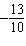Name:    Quiz B.1-B.3

Multiple Choice
Identify the choice that best completes the statement or answers the question.

Tell which number is greater.

1.

–15, –10
 a. 15 c. –15 b. 10 d. –10

2.

–6, 13
 a. –13 c. –6 b. 6 d. 13

3.

18, –14
 a. 18 c. –18 b. 14 d. –14

Simplify the expression.

4.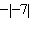a. 7 c. –7 b.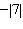d.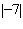Use a number line to find the sum or difference.

5.

–6 + 2
 a. 4 c. –8 b. –4 d. 8

6.

10 – 23
 a. 33 c. –13 b. 13 d. –33

Use a number line to evaluate the expression.

7.

–5 – 8 + 9
 a. 12 c. 4 b. –4 d. –22

8.

7 + 2 – 6
 a. 3 c. 15 b. –11 d. –1

9.

–7 – 5 – 9
 a. –11 c. –3 b. –21 d. –7

Find the median of the data.

10.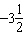,,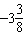,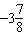,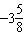a.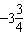c.b.d.11.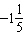,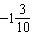,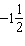,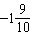,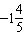a.c.b.d.Numeric Response

Find the absolute value of the integer.

1.

28

2.

–14

Find a fraction or a mixed number that is between the two numbers.

1.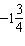,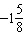Which number is greater? Explain.

2.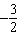,